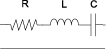seriesRLC

Create two-port series RLC circuit

Description

Use the seriesRLC object to create a circuit representing as a resistor, inductor, and capacitor connected in series. The series RLC circuit object is a two-port network as shown in this circuit diagram.You can also use the seriesRLC object to model a series RLC circuit in cascaded stages using an rfbudget object or the RF Budget Analyzer app.

Creation

Description

rlc = seriesRLC creates a default series RLC object.

example

rlc = seriesRLC(Name,Value) sets Properties of the serisRLC object using one or more name-value arguments. For example, rlc = seriesRLC('R',80) creates a series RLC object with the resistance set to 80 ohms. Properties you do not specify retain their default values.

Properties

expand all

Name of the series RLC circuit, specified as a string scalar or a character vector.

Example: rlc = seriesRLC('Name','RLCseriescircuit') creates a series RLC circuit called RLCseriescircuit.

Resistance value of the series RLC circuit, specified as a positive scalar in ohms.

Example: rlc = seriesRLC('R',75) creates a series RLC circuit with the resistance set to 75 ohms.

Inductance value of the series RLC circuit, specified as a positive scalar in henries.

Example: rlc = seriesRLC('L',1e-6) creates a series RLC circuit with the inductance set to 1e-6 henries.

Capacitance value of the series RLC circuit, specified as a positive scalar in farads.

Example: rlc = seriesRLC('C',2.2e-9) creates a series RLC circuit with the capacitance set to 2.2e-9 farads.

Number of input and output ports of the series RLC circuit, returned as a positive scalar.

Terminals of the series RLC circuit, returned as a cell array.

Object Functions

 sparameters Calculate S-parameters for RF data, network, circuit, and matching network objects groupdelay Group delay of S-parameter object or RF filter object or RF Toolbox circuit object noisefigure Calculate noise figure of transmission lines, series RLC, and shunt RLC circuits

Examples

collapse all

Create a series RLC circuit with the specified parameters.

rlc = seriesRLC('R',2e3,'L',40e-3,'C',1e-6);

Calculate the S-parameters of the series RLC circuit at 1 GHz.

spar = sparameters(rlc,1e9)
spar =
sparameters: S-parameters object

NumPorts: 2
Frequencies: 1.0000e+09
Parameters: [2x2 double]
Impedance: 50

rfparam(obj,i,j) returns S-parameter Sij

Calculate the noise figure of the series RLC circuit at 1 GHz.

nf = noisefigure(rlc,1e9)
nf = 16.1278